am
🧠

# Logic Gates

• Performs a basic operation on electrical signals
• Accepts one or more inputs and produces a single output signal
• Easiest gates to make are NOT, NAND, and NOR gates
•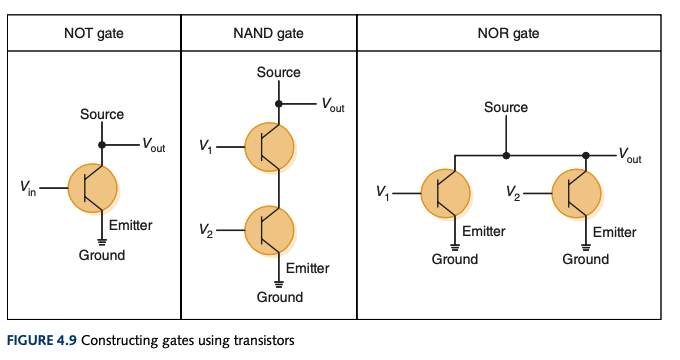## NOT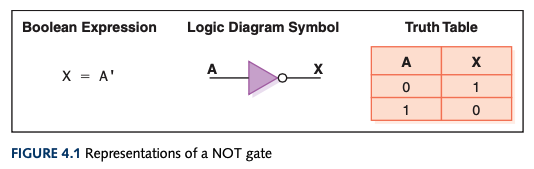• Accepts an input
• Output is inverted of input
• An inverter
• Boolean algebra:
• A'
• The mark comes after a value being negated
• Sometimes shown as horizontal bar over value being negated
• Logic diagram:

A Output
0 1
1 0

## AND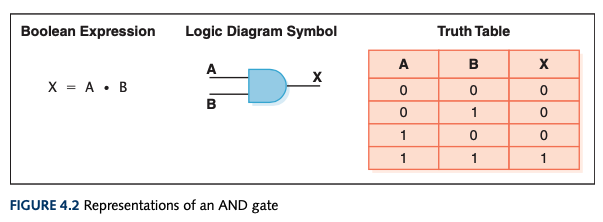• Accepts two inputs
• Outputs 1 if both inputs are 1
• Outputs 0 if either input is 0
• Boolean algebra:
• A • B or A * B or AB or A ^ B

A B Output
0 0 0
0 1 0
1 0 0
1 1 1

## OR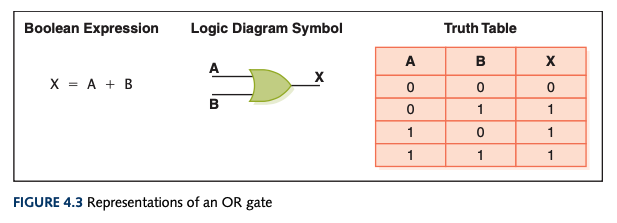• Accepts two inputs
• Outputs 0 if both inputs are 0
• Outputs 1 otherwise
• Boolean algebra:
• A + B or A v B

A B Output
0 0 0
0 1 1
1 0 1
1 1 1

## XOR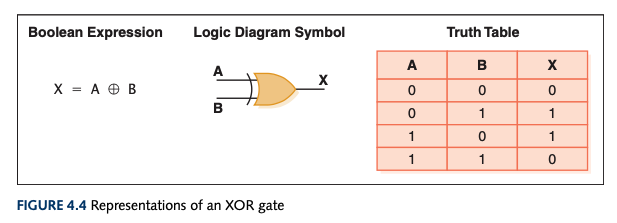• Exclusive OR
• Only differs from an OR if both inputs are 1
• "When I say or, I mean one or the other, not both."
• Accepts two inputs
• Outputs 0 if the two inputs are the same
• Outputs 1 otherwise
• Boolean algebra:
• Circle with a plus in it

A B Output
0 0 0
0 1 1
1 0 1
1 1 0

## NAND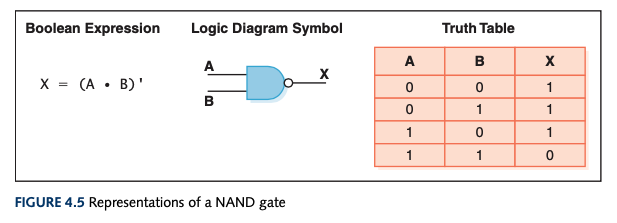• NOT + AND
• It's an AND gate that gets inverted
• Accepts two inputs
• Outputs 0 if both are 1
• Outputs 1 otherwise
• Boolean algebra:
• (A • B)'

A B Output
0 0 1
0 1 1
1 0 1
1 1 0

## NOR• NOT + OR
• It's an OR gate that gets inverted
• Accepts two inputs
• Outputs 1 if both input is 0
• Outputs 0 otherwise
• Boolean algebra:
• (A + B)'

A B Output
0 0 1
0 1 0
1 0 0
1 1 0

Computer Science